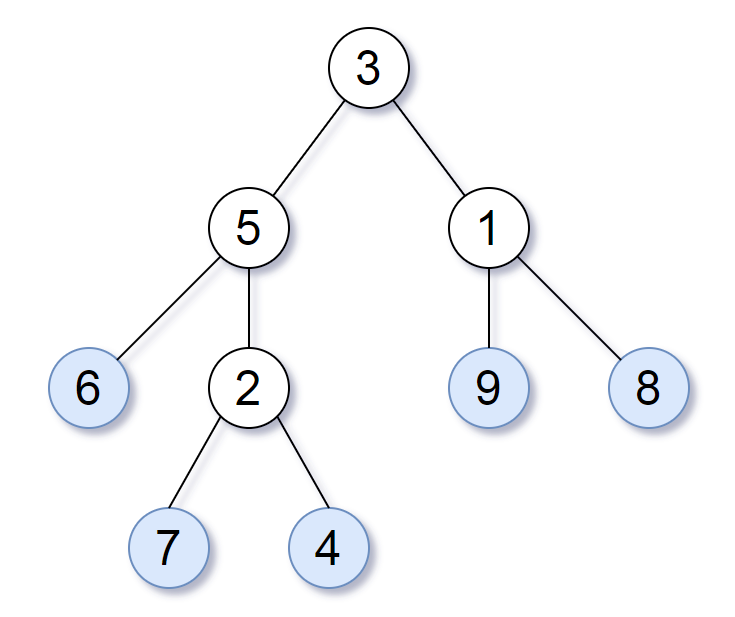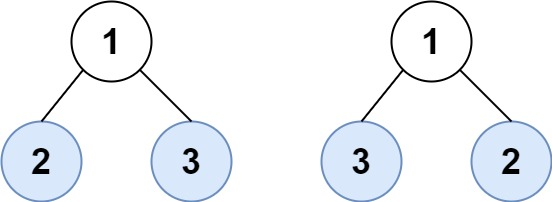# 872. Leaf-Similar Trees

Easy
Consider all the leaves of a binary tree, from left to right order, the values of those leaves form a leaf value sequence.For example, in the given tree above, the leaf value sequence is `(6, 7, 4, 9, 8)`.
Two binary trees are considered leaf-similar if their leaf value sequence is the same.
Return `true` if and only if the two given trees with head nodes `root1` and `root2` are leaf-similar.
Example 1:Input: root1 = [3,5,1,6,2,9,8,null,null,7,4], root2 = [3,5,1,6,7,4,2,null,null,null,null,null,null,9,8]
Output:
true
Example 2:Input: root1 = [1,2,3], root2 = [1,3,2]
Output:
false
Constraints:
• The number of nodes in each tree will be in the range `[1, 200]`.
• Both of the given trees will have values in the range `[0, 200]`.

### 解題

Runtime: 0 ms, faster than 100.00%
Memory Usage: 2.6 MB, less than 35.29%
/**
* Definition for a binary tree node.
* type TreeNode struct {
* Val int
* Left *TreeNode
* Right *TreeNode
* }
*/
func leafSimilar(root1 *TreeNode, root2 *TreeNode) bool {
arr1 := getLeaf(root1)
arr2 := getLeaf(root2)
return reflect.DeepEqual(arr1, arr2)
}
func getLeaf(root *TreeNode) []int {
leaves := make([]int, 0)
var recursive func(root *TreeNode)
recursive = func(root *TreeNode) {
if root.Left==nil && root.Right==nil {
leaves = append(leaves, root.Val)
}
if root.Left!= nil {
recursive(root.Left)
}
if root.Right!= nil {
recursive(root.Right)
}
}
recursive(root)
return leaves
}